# SSAT Middle Level Math : Operations

## Example Questions

← Previous 1 3 4 5 6 7 8 9 10 11 12

### Example Question #1 : Operations

Which of the following is NOT the same as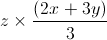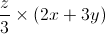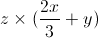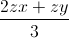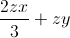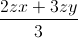Explanation:

The answer shows that the 3 in front of thehas been cancelled, but not removed from the denomitator for the.

### Example Question #1 : Variables

Simplify: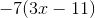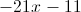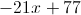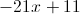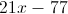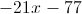Explanation:

Apply the distributive property: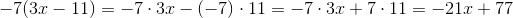### Example Question #1 : How To Multiply Variables

Simplify: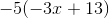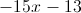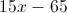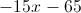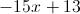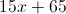Explanation:

Apply the distributive property: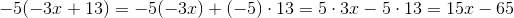### Example Question #4 : Operations

Simplify: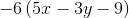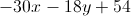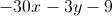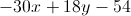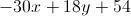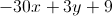Explanation:

Apply the distributive property: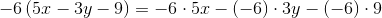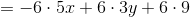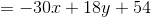### Example Question #1 : Variables

Simplify: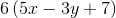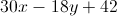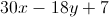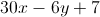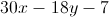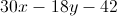Explanation:

Apply the distributive property: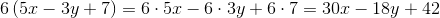### Example Question #6 : Operations

Shaun has twice as much money as Jessica. Jessica has one third as much money as Chris. Shaun has half as much money as Carmen. Who has the most money?

Carmen

Both Shaun and Carmen

Shaun

Jessica

Chris

Carmen

Explanation:

In order to figure out who has the most money, we must organize the data we have. Since Shaun has more money than Jessica, let us use Jessica as our baseline. So, if the money that Jessica has is represented by, the money Shaun has will be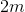because he has twice as much money as Jessica.

Jessica =Shaun =Next, we know that Jessica has one third as much money as Chris. In other words, Chris has three times as much money as Jessica. This can be represented by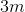. We then learn that Shaun has half as much money as Carmen or, in other words, Carmen has two times the amount of money Shaun has. Since Shaun hasdollars, that must mean Carmen has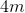dollars because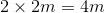. So, by comparing everyone side-by-side, we can see that Carmen has the most money as she has four times the amount of money that Jessica has.

Jessica =Shaun =Chris =Carmen =### Example Question #7 : Operations

Given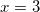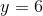, and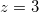,  compute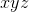.Explanation:refers to the product of the three variables: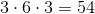.

### Example Question #5 : Multiplying And Dividing Polynomials

Simplify: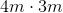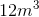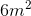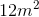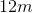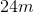Explanation:

To solve, you can use the commutative and associative properties of multiplication to group like-terms together.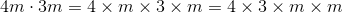The 4 and 3 should be first multiplied, resulting in 12.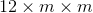Nextshould be multiplied by, giving us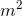.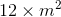12 timesis equal toTherefore, the correct answer is.

### Example Question #8 : Operations

Simplify: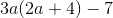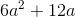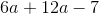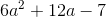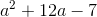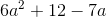Explanation:

When simplifying this expression, the first step is to apply the distributive property.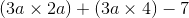Next, we assert whether the expression can be reduced further. It cannot, as there are no like-terms to combine.

Therefore, the correct answer is.

### Example Question #9 : Operations

Suppose you know the values of all variables in the expression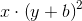and you want to evaluate the expression.

In which order will you carry out the operations?